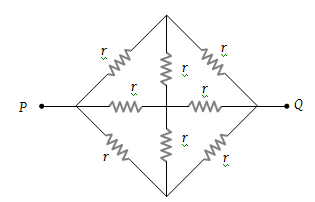The equivalent resistance between the points P and Q in the network given here is equal to (given $r=\frac{3}{2}\Omega$)(1) $\frac{1}{2}\text{\hspace{0.17em}\hspace{0.17em}}\Omega$

(2) 1 Ω

(3) $\frac{3}{2}\text{\hspace{0.17em}\hspace{0.17em}}\Omega$

(4) 2 Ω

Explanation is a part of a Paid Course. To view Explanation Please buy the course.

Difficulty Level: